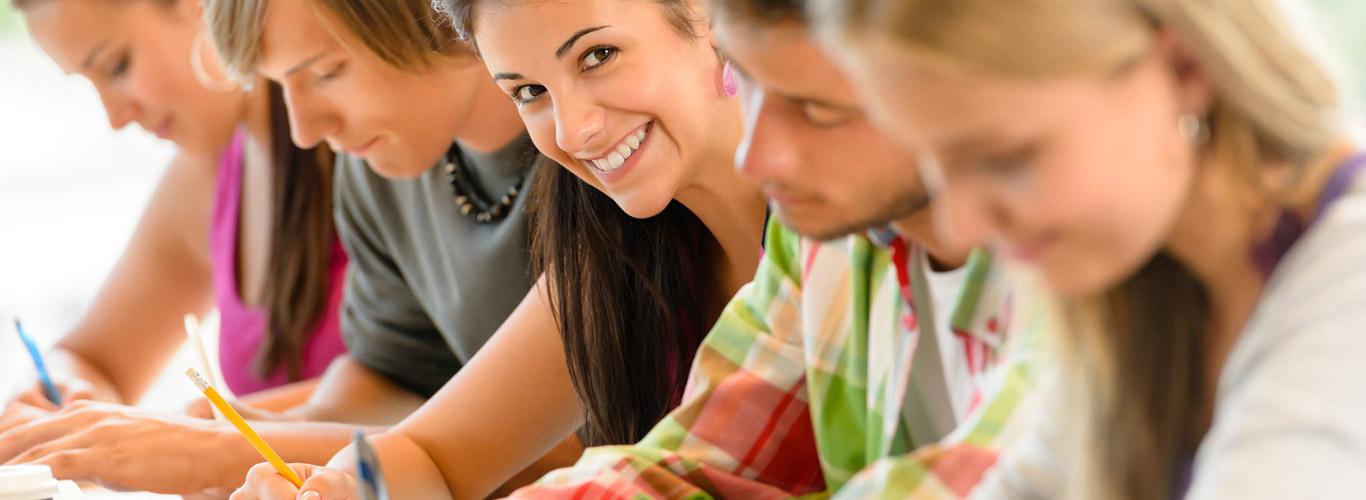## Science-AW462

Science-AW462 Online Services   QUESTION 1   a) A kilogram of water at 20 ºC is placed in the freezer and its temperature is brought to 0 ºC. Given: Specific heat of water = 4190 J/kgK; Latent heat of fusion of water= 333000 J/kg   Calculate,   i) How much thermal energy was transferred, if n...

## Physical and Chemical Change Analysis With Solution

Physical and Chemical Change Analysis With Solution   1.A water sample has the following composition data: CaCo3= 100 ppm, MgCO3= 42 ppm, MgCl2= 95 ppm, CaSO4= 136ppm, MgSO4= 120ppm, CO2= 44ppm, NaAlO2= 164ppm.   Calculate the quantity of lime and soda required for softening of one million liter of water.     2.State whether each of the following r...